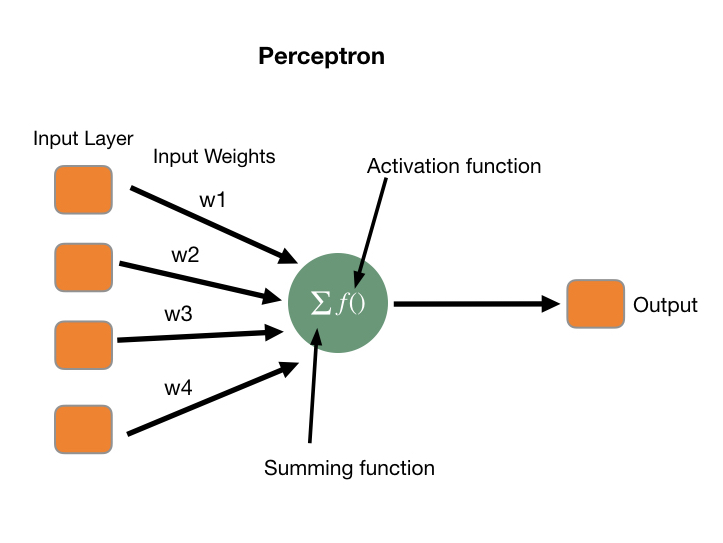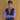# Calculate outputs of layers in neural networks using numpy and python classes

June 17, 2020

• Introduction
• In the last article we covered how dot product is used to calculate output in a a neuron of a neural network.
• For just a brief recap here are the essential parts of a node in neural network• We calculate the output using the formula• In today’s article, we are going to understand how to calculate the output value in neural networks using numpy and python class.
• Let’s say we have a set of inputs in for our neural network as follows:
``````# Implement multilayer neural network using numpy
import numpy as np
#Input Values
X = [[1,2,5,7],
[0.5,0.6,0.7,0.8],
[1.2,2.1,2.2,1],
[1.3,4.5,6.7,8.9]
]``````
• First step we do is initialise a class in Python for our layer as shown below:
``````  #Class for creating layer
class Layer_Dense:
def __init__(self, n_inputs, n_neurons):
self.weights = 0.10 * np.random.randn(n_inputs, n_neurons)
self.biases = np.zeros((1, n_neurons))  ``````

In line no 2 we initialised a class for our layer. The `__init__` method helps us to initialise the attributes in a class.
The first variable we define in the init method is weights array with random values. We make use of np.random method to initialise it with random values.
Next we initialise the bias values in an array with zero values initially.

• Now we can calculate the output of the layer with the formula shown above as follows:
``````     def forward(self, inputs):
self.output = np.dot(inputs, self.weights) + self.biases``````
• We can then pass the output from one layer to another as followed:
``````#Sample layer example
layer1 = Layer_Dense(4,5)
layer2 = Layer_Dense(5,2)

layer1.forward(X)
# print(layer1.output)
layer2.forward(layer1.output)
print(layer2.output)``````

You can find complete code for the example here: Github

• This covers a short introduction on calculation of layer outputs in numpy. In the next article we will see how to combine this with an activation function to get a basic working neural network.
• References: Neural Networks from Scratch by SentdexBlog by Saurabh Mhatre
Follow me on Twitter